### Home > CAAC > Chapter 6 > Lesson 6.2.5 > Problem6-86

6-86.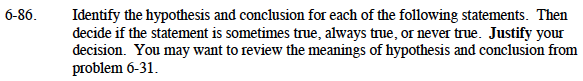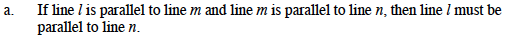Write the hypothesis and conclusion.

Hypothesis: line l is parallel to line m and that line m is parallel to line n.
Conclusion:line l must be parallel to line n.

Draw lines l, m, and n, so line l is parallel to line n and line m is parallel to line n.
Is there more than one way to draw this situation?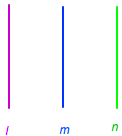Sometimes true, because line l and line n could coincide (overlap each other).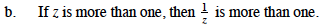Write the hypothesis and conclusion.

Hypothesis: z is more than one

$\text{Conclusion: }\frac{1}{z} \text{ is more than one.}$

Try an example, using a value greater than 1 for z.

z = 3 3 > 1

$\frac{1}{z}=\frac{1}{3} \text{ is } \frac{1}{3} >\text{ or }<1\text{?}\frac{1}{3}<1$

$\text{never true because }\frac{1}{x}<1\text{ if }x>1$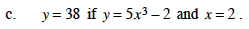Write the hypothesis and conclusion.

Hypothesis: y = 5x3 − 2 and x = 2
Conclusion: y = 38

Substitute x = 2 into the equation and solve for y.
Find y:
y = 5(2)3 − 2
y = 5(8) − 2
y = 40 − 2
y = 38

Always true